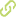# Semi-analytical derivation of approximate non-linear eigenvalues and eigenvectors for general non-linear MDOF systems•
• Overview
•
• Identity
•
•
• View All
•

### Abstract

• This work introduces a novel theoretical formulation for the evaluation of approximate eigenvalues and eigenvectors for general non-linear MDOF systems using the so-called "approximate non-linear mode evaluation" (ANME) method. The approach is based on analytically derived quasi-linear expressions which relate the change in modal parameters to physical non-linear elements that can be added anywhere in the system. Subject to assuming that the change in the mode shape is small, expressions for non-linear eigenvalues and eigenvectors become fully determined for a general MDOF system, even within typical experimental constraints. The errors arising from this assumption can be minimized via an iterative procedure. Preliminary results indicate that the rate of convergence is quite fast for systems with medium to high damping but more effort is required for lightly damped systems. The derived expressions provide a theoretical basis for observations made by previous researchers from an inspection of their experimental and/or numerical results: these indicate that an invariant relationship exists between a non-linear eigenvalue and its associated modal response. © 2007 Elsevier Ltd. All rights reserved.

### Publication date

• January 1, 2009